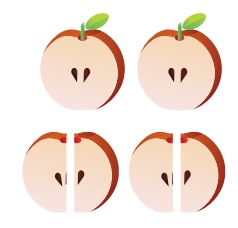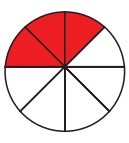Home | | Maths 4th Std | To identify the notation of fractions

# To identify the notation of fractions

Denominator is the total number of parts that make up a whole. Numerator represents the number of equal parts on a whole.

To identify the notation of fractions:

Introduction:

Ramu and Akil playing with their friends Rangan and thangam. Ramu’s mother had an apple, so she called Ramu and Akil. She made it into two halves and gave it to them. She totaly forget Rangan and thangam. So she recollect the two pieces of apple from (her son) them. Then she made it as four equal parts and give it to them.An apple fruit brought by Ramu’s mother = 1

Here 1 means whole

First one half given to Ramu = 1/2

Other half given to Akil = 1/2

If a whole is divided into two parts then its denominator is 2.

A whole is divided into two equal parts.

The divided parts is denominator.

Here ½ which means 2 is denominator and 1 is numerator.

When Ramu’s mother Collected the two equal parts of an apple from her sons and sliced it once again into four equal parts.

Four equal parts of an apple (the whole)Denominator is the total number of parts that make up a whole.

Numerator represents the number of equal parts on a whole.

A part given to Ramu = 1/4

A part given to Akil = 1/4

A part given to Rangan = 1/4

A part given to thangam = 1/4

If a whole is divided into four equal parts then its denominator is 4.

In 1/4, 4 means denominator and 1 means numerator.

EXAMPLE

There are 10 Guava’s in a basket which are equal sized.

Here the 10 Guava’s makes a whole.So it is the denominator (10 Guava’s = whole)

If we take 4 Guava’s = 4 / 10Now 10 means denominator and 4 means numerator.

Remaining fruits in the basket = 6/10Now 10 means denominator and 6 means numerator.

Numerator and Denominator of different Shapes.The square is divided into four equal parts 4 equal parts said to be denominator, in this four parts one portion (part) is coloured, so the coloured one portion is numerator. Now we can write this as 1/4 .This circle is divided into eight equal parts.

So its denominator is 8.

In the 8 parts coloured 3 parts are said to be numerator.

so its numerator is 3.

Now we can write it as 3/8

3 Slash 8 = 3/8 = The number above slash is Numerator/ The number below slash is DenominatorTags : Fraction | Term 2 Chapter 6 | 4th Maths , 4th Maths : Term 2 Unit 6 : Fraction
Study Material, Lecturing Notes, Assignment, Reference, Wiki description explanation, brief detail
4th Maths : Term 2 Unit 6 : Fraction : To identify the notation of fractions | Fraction | Term 2 Chapter 6 | 4th Maths